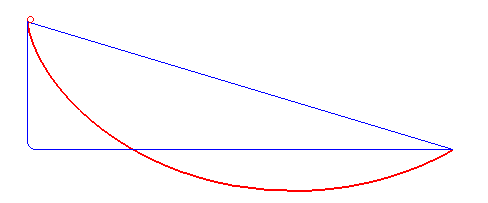## Math teaching strategies #6: Teaching a new concept using a common sense name

Here’s an example of the problem.   A brachistochrone (pictured above) is a curve between two points along which a body can move under gravity in a shorter time than for any other curve. It is the same curve as a cycloid, but just hanging downward.   A cycloid is the path traced by a point on a wheel as the wheel rolls, without slipping, along a flat surface. The standard parametrization is x = a(t – sin t),y = a(1 – cos t), where a is the radius of the wheel.Introducing this concept and the term brachistochrone at the same time would be designed so the teacher can use brachistochrone and its concept in instruction.  However, because the term is new and the concept is also new, when the teacher uses the new term during later instruction, the students will have difficulty bringing the concept into their minds. “So a brachistochrone has some other cool properties. What’s the primary thing we know about the brachistochrone?”

Instruction not working.  If you watch instruction where the term and the concept have been taught simultaneously, confusion ensues when the teacher uses the term.  You’ll see students looking away as they try to bring up the explanation of that weird new term in their memory (see the pictured example?).  Sometimes the teacher will notice this and give a thumbnail definition or example of the term, and the students will then remember.  However, the teacher should then realize that the concept was not connected to the new vocabulary term.

## Math teaching strategies: Teach new concepts using a common sense term at first.

When a new vocabulary term is used to introduce a new concept, students will need a lot of practice with recalling the term and the definition before it can be used in instruction.  On the other hand, students can quickly understand and use new concepts and ideas in math if they don’t have to learn a new word for it.  Using a common sense term, the idea or concept can be worked with, the implications studied and it can be applied to real world problems almost immediately.  Students can later quite easily learn proper vocabulary terms for concepts they understand and recognize.  Here’s an example.

The “shortest time curve.”  It is more efficient and effective to teach the concept first and use a common sense term for it.  I would call a brachistochrone the “shortest time curve.”**  Instruction would proceed with the, “Do you remember that ‘shortest time curve’ we talked about last week?”  Students would easily be able to remember it.  Instruction would go like this: “So ‘the shortest time curve’ has some other cool properties. What’s the primary thing we know about the ‘shortest time curve’?”  Students would easily be able to answer this question.

Then after students have worked with the concept of “the shortest time curve” for a couple of weeks, you can add the vocabulary term to it. “By the way, the proper mathematical name for “the shortest time curve” is called a brachistochrone. Isn’t that cool?”  Students will want to learn its proper name as a point of pride about knowing this fancy term for a concept they already “own,” rather than a point of confusion.

**Actually that’s what brachistochrone means in Greek: brakhistos, meaning shortest and khronos meaning time.